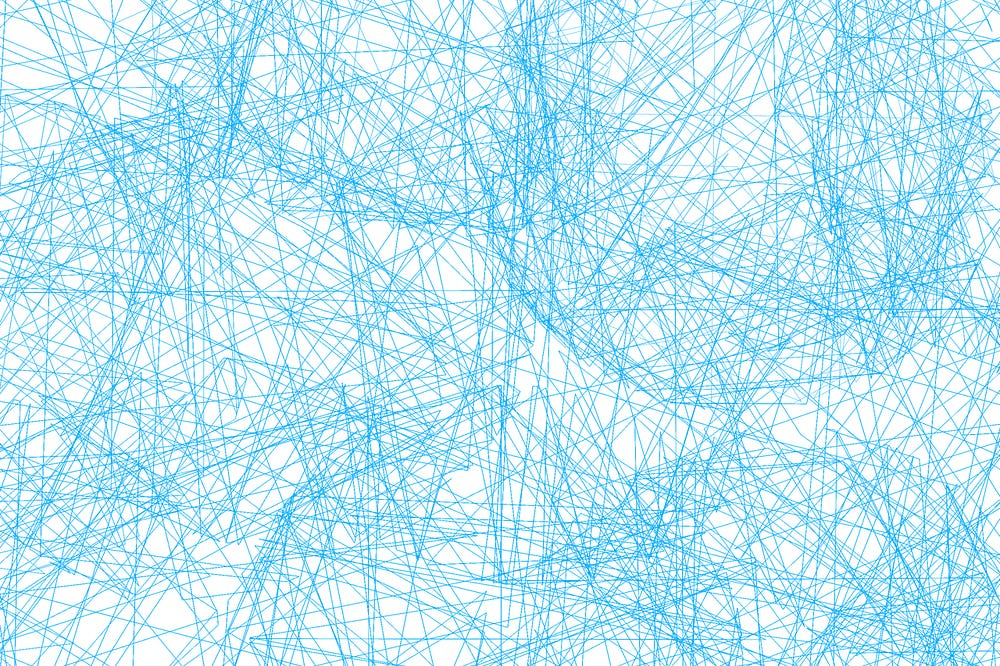Deep learning for recommender systems

In my past article on latent collaborative filtering, we used matrix factorization to recommend products to users. The input for that algorithm was UserItemRating matrix R. This matrix contains all the ratings of all the products given by all the users. This is the same matrix we are going to use to train our neural network. If we want to take this as input for neural network then there becomes a problem because it is integer identifier for user and items. For example, say the following users are tracking following items:

UserId MovieId
455 344
345 433
23 425
567 753

If we feed this into neural network then we don’t get anything. So the idea here is to find a good representations of them. The same problem arises in neural nets when we deal with text tokens in NLP, categorical variables in other ML models like tags, category etc. Say ‘s’ be the symbol in the vocabulary V. Then for a word ‘love’ in the dictionary, we can represent as :

one-hot-encoding(‘love’) = [ 0, 0, 0, 0 …… 1 …… 0]

Here this vector is very sparse and has huge dimension. Also another problem is that the distance between the vectors are equi-distance. Meaning that love and hate are in same distance as love and peace. So this does not capture the meaning of concept clearly.

Embedding

Instead we want to encode the vectors as continuous values in a lower dimensional space. This is known as embedding. E.g

embedding(‘love’) = [3.23, -4.5, 5.2, ……. 9.3]

We can quantify this my using distance metrics like Euclidean distance or Cosine similarity between the vectors. If you want to learn more about the distance metrics here is a good article.

1. It is continuous and dense.
2. Embedding metric can capture symmetric distance.

Another way you can see the embedding is as the linear layer of neural network typically as the input layer that maps one-hot representation into the continuous space.We can achieve this by multiplying one hot-representation with embedding matrix W ∊ R n x d.

embedding(s) = onehot(s).W

We initialize W randomly in the start and entries of this matrix are tunable. They are also known as embedding parameters.

In Keras:

So now we have the output of embedding that can be fed into our model and define loss function depending on the target we want to predict. We then get the tunable architecture and we use gradient descent to adjust the parameters of embedding.

Now our initial problem was to predict ratings for product j by user i. In my previous post I have talked about matrix factorization way of doing so. Now the concept is similar but we input the embedding vectors for our items and products. Then we take dot product of them and minimize the loss function.

So the concept looks pretty similar but what is the advantage of doing this in neural network way?

1. Instead of just using dot product as the interaction(rating) we can use multi layer perceptron with many fully connected layers to calculate the rating or interaction

First, we concatenate the embedding then feed into the neural network and this will give us the ratings as the output. And we use the same loss function to minimize the errors.

2. The size of embedding can also be different meaning that we can have items lot more than users. We can also concatenate the metadata information into the neural network. For instance if some metadata have categorical variables like director of movie, we can define new embedding for directors or new embedding for movies and so on.

So we have many embeddings as the input rather than single matrix factorization model.

But if we don’t have an explicit feedback from users, we cannot use the regression loss function like before. We should use another architecture also known as triplet architecture.

In this architecture, we have a user i and he has watched movie j. Then we also pick up another movie k at random from the database and it is very likely that user has not seen the movie past or will not see in the future because there are lot of movies that are negative(meaning that user might not be interested in watching). So we contrast negative movie with positive movie for a given user. So we compute the two interactions by taking the dot product and we compute difference between them. And we make sure that the positive interaction between user and positive movie is larger than the user and a random negative movie. Then we minimize the loss and maximize the difference. And at the end we tune the embedding and come up with good recommendations.

The V embedding for positive and negative items is a same matrix, we just take different rows in the same matrix. So we train with same model parameters. These type of networks that use the same model parameters are known as Siamse networks.

YouTube uses the same concept of learning embedding parameters to recommend videos to users.

Here the metadata are geographical information, age, gender and more. We feed these embedding into the neural networks and the serving selects top N nearest neighbors and then softmax calculates the probability of the user watching that video. Then the results are sorted in descending order and presented to user.

Conclusion

1. Deep learning boosts performance of recommendation systems.
2. Architectures like CNN are best for content based feature learning and for cold start problems.
3. RNN are best for sequential recommendations

Source: Deep Learning on Medium